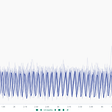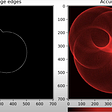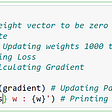# RL — Value Fitting & Q-Learning

We can learn the value function and the Q-value function iteratively. However, it cannot scale well to large state space. In practice, we don’t have enough memory for all the states. But we can approximate it through the model fitting. The most common method is to use a deep network as a function approximator.

# Value Fitting

If the state space is continuous or large, it is not possible to use a large memory table to record V(S) for every state. However, like other deep learning methods, we can create a function estimator to approximate it.

where y is our target value.

There are many ways to establish the target value and we will discuss them in the next few sections.

Monte-Carlo Method

Monte-Carlo method answers questions by repeat random sampling. For example, we can compute the reward of an episode by sampling actions from a policy.

By continuous sampling, we apply supervised learning to train Φ to match targets. For example, the rewards (or penality) from San Francisco to San Diego is computed as -10. Therefore, V(SF) equals -10 in this episode.

Monte-Carlo method is non-biased. We play out the whole episode to find the exact reward of an action sequence. However, the Monte-Carlo method has high variance. If the policy or the model is stochastic, the sampled actions are different and lead to different rewards in different episodes.

A stochastic policy is often used in RL to explore space and to smooth out the rewards for a better gradient behavior. However, for each run, the trajectory is different.

To fit the value function modeled by Φ, we collect samples and use supervised learning to train the model.

In many RL tasks, a small change in action can lead to a very different result. Such a large variance in rewards can destabilize the training badly.

Temporal difference TD

Let’s assume that we bootstrap the value function from San Francisco to San Diego as (in a real-life example, we usually initialize V with all zero):

Instead of the Monte-Carlo method, we can use the temporal difference method TD to compute V. We take k-steps and combine the observed rewards with the V value for the state landed after k-steps. For example, in a 1-step TD lookahead, the V(S) of SF equals the rewards from SF to SJ plus V(SJ).

First, we observe the rewards for taking each action and then use a 1-step lookahead to update V. The diagram below demonstrates how V(S) is updated after taking three consecutive actions.

This scheme has a lower variance because we take just one action in finding the target for V. However, at least in the early training, it is highly biased as the V values are not accurate. As we progress, the bias in V will lower.

Here, we observe the reward of action and combine it with the fitted value of the next state to train Φ.

Here is the result with a 2-step lookahead.

As the number of lookahead increases, it is getting closer to the Monte-Carlo method.

TD(λ)

We don’t need to settle with the Monte-Carlo or TD method exclusively. Instead, in TD(λ), we combine all k-step lookaheads (from k=1 to the end) with weights

and use the combined results as the total rewards.

λ is a hyperparameter that can be tuned to control whether we want to have higher biased or higher variance during the training. When λ=0, it is TD, and when λ=1, it is the Monte-Carlo method.

# Q-learning

To use the value function in deciding our actions, we need to know the model, (knowing the state transition after taking an action). Otherwise, even we know what is the best future state, we don’t know which action leads to it. For chess, the model is defined by the rule of the game. But for many problems, we don’t know the model.

Without a model, we can apply Q-value learning. One of the most important methods for Q-value learning is Q-Learning.

Q-learning is an online Q-value function learning:

1. We sample action from a state using epsilon greedy (SA).
2. We observed the reward and the next state (SARS’).
3. We use the Q-value function to find the action a’ with the maximum Q-value (SARSA Max).

In step 3, we pick the maximum Q-value for a more deterministic path in helping the algorithm to converge better. Here is the flow:

It can be explained mathematically as:

Algorithm

If the combination of s and a is manageable, we can store all the Q values in a table and use the following algorithm to compute Q.

Otherwise, we can use a deep network parameterized by Φ to approximate Q.

Here is the algorithm:

In the example above, we use an off-policy exploration, like epsilon-greedy, to select the action to be taken from s. Epsilon-greedy policy balances between exploration and exploitation. In general, it explores more promising actions more frequently but yet allows some chance to explore less promising actions. In early training, Q is initialized with 0. Hence, there is no specific action that stands out. Early training will explore randomly. As more actions are sampled, we know better about Q. Action with higher Q will be selected more. The Epsilon-greedy policy allows us to select more promising actions and yet allows some randomness for exploration. But such exploration will decrease as the training progress.

Pseudocode

This is the pseudocode for Q-learning using a function approximator.

• It is an off-policy method that utilizes off-policy samples. This improves sample efficiency (the number of samples needed to optimize a policy).
• It has a lower variance comparing with policy gradient methods which usually have a high variance policy gradient.

# Deep Q-learning DQN with the replay buffer and the target network

Q-Learning with function approximation suffers a few major issues:

• Input samples within a trajectory are highly correlated. This is bad for supervised learning. It amplifies changes and noises.
• Our target value keeps changing.

Both destabilize training.

The details of the problem can be found here. But to make training samples independent of each other, we store the latest samples in a replay buffer and randomize them for training. This makes samples less correlated within a training batch. To avoid a moving target, we also delay the Q-value network updates so the target does not change constantly. This topic deserves a whole new article so we will briefly list the algorithm for reference only.

# Partially Observable MDP (POMDP)

Value-Learning computes the expected rewards from a state. Nevertheless, a state may not be fully observable. For example, the view of a pedestrian may be blocked by a car. The blocked information may change the value function completely if the pedestrian is observeded.

In value-learning in a POMDP, we may use a sequence of states rather than a single state to compute the value. In the example above, we pass sequential image frames to a CNN to extract features and use an RNN to record all the history into an RNN state. We then use the RNN state to compute the value function.

Let’s summarize some of the limitations of value learning.

• Value iteration, using value function V, requires the model P.
• Value iteration and Q-Learning require a function estimator to scale the solution to continuous or large state space.

Pro for value learning, Q-Learning, TD learning & DQN:

• They reuse old sample data or results. This improves data efficiency.
• Compared with PG or the Monte-Carlo methods, these methods have low variance and less volatile gradient change. The training can be more stable.

Con for value learning, Q-Learning, TD learning & DQN::

• A non-linear function estimator does not have any convergence guarantee.
• Value Learning does not optimize the policy directly. Optimizing a policy is not necessarily the same as value learning. In-accuracy may introduce.
• Finding the optimal action using Q-value requires finding the maximum Q effectively. This may not be easy for continuous or large action space. Complex optimization techniques may be required to optimize:

# Value learning v.s. Policy Gradient

There is no definite answer on whether we should use value learning methods or PG methods. For value learning methods, there are no guarantee for the model to converge and methods like DQN may have a lot of hyperparameters to tune. When in doubt, some researchers may suggest PG methods as an easier start. PG methods focus on optimizing a policy. Since it operates in the policy space directly, the solution is easier to interpret and may manage to find a good enough policy in solving a complex problem. And even though PG methods tend to converge to a local optimal. This local optimal is usually good enough in practice.

Nevertheless, PG methods have high variance. If the reward function has a steep curvature, it can hurt the training progress badly with a small innocent move. Value-learning is more sample efficient and favors problems that are expensive to collect samples. But, there is no theoretical justification on which method is better. Many differences are caused by how patient the models are tuned and trained. In addition, methods are often improved to address their shortcomings.

The choice is mainly based on issues related to sample efficiency, variance, and convergence. But it is hard to find a golden rule. It is easier to sample moves in GO game. Yet, AlphaGo Zero, one of the hardest RL problems, beats GO masters with mostly value learning. In practice, many solutions are blended together with value learning and policy gradient to address their weakness.

--

--

--

## More from Jonathan Hui

Deep Learning

Love podcasts or audiobooks? Learn on the go with our new app.

## Making better cheese with IoT and ML## Hough Circle Transform in OpenCV## Dstl Satellite Imagery Competition, 3rd Place Winners’ Interview: Vladimir & Sergey## Using Machine Learning to Predict Subscription to Bank Term Deposits for Clients with Python…## Prediction of Loan Approval with Machine Learning## Gradient Descent Implementation in Python## A MACHINE LEARNING PERSPECTIVE INTO NEW BEGINNINGS.Deep Learning

## Data Driven (Reinforcement Learning based) Control## Building A Virtual Self-Driving Car Using Deep Q-Learning## Reinforcement Learning